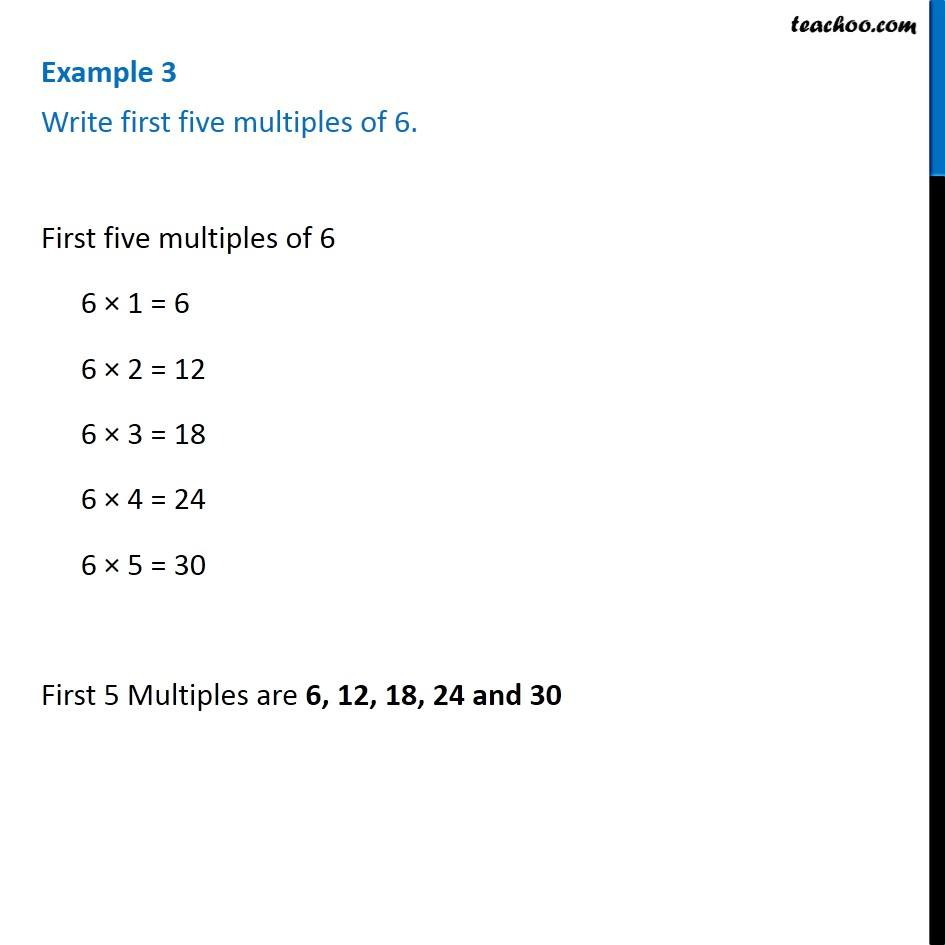1. Chapter 3 Class 6 Playing with Numbers
2. Concept wise
3. Factors and Multiples

Transcript

Example 3 Write first five multiples of 6. Multiples of 6 will be numbers will which be in its table Thus, First five multiples of 6 6 × 1 = 6 6 × 2 = 12 6 × 3 = 18 6 × 4 = 24 6 × 5 = 30 First 5 Multiples are 6, 12, 18, 24 and 30

Factors and Multiples